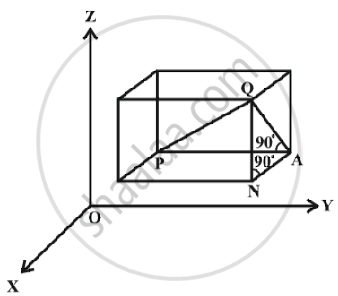# Three - Dimensional Geometry - Distance Between Two Points

#### description

• Distance Between Two Points in 3-D Space

#### notes

Let P(x_1, y_1, z_1) and Q ( x_2, y_2, z_2) be two points referred to a system of rectangular axes OX, OY and OZ. Through the points P and Q draw planes parallel to the coordinate planes so as to form a rectangular parallelopiped with one diagonal PQ.Fig.Now, since ∠PAQ is a right angle, it follows that, in triangle PAQ, PQ^2 = PA^2 + AQ^2                                       ... (1)
Also,  triangle ANQ is right angle triangle with ∠ANQ a right angle.
Therefore       AQ^2 = AN^2 + NQ^2     ... (2)
From (1) and (2), we have

PQ^2 = PA^2 + AN^2 + NQ^2

Now    PA = y_2 – y_1, AN = x_2 – x_1 and NQ = z_2 – z_1

Hence PQ^2 = (x_2 – x_1)^2 + (y_2 – y_1)^2 + (z_2 – z_1)^2

Therefore PQ = sqrt((x_2-x_1)^2 + (y_2-y_1)^2+(z_2-z_1)^2)

This gives us the distance between two points (x_1, y_1, z_1) and (x_2, y_2, z_2).

In particular, if x_1 = y_1 = z_1 = 0, i.e., point P is origin O, then

OQ = sqrt(x_2^2+y_2^2+z_2^2) , which gives the distance between

the origin O and any point Q (x_2, y_2, z_2).

If you would like to contribute notes or other learning material, please submit them using the button below.

### Shaalaa.com

Distance between Two points [00:11:27]
S
0%